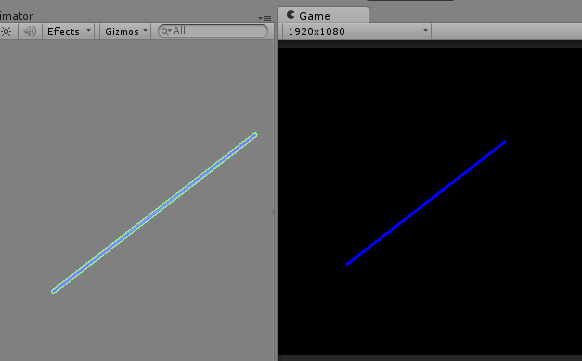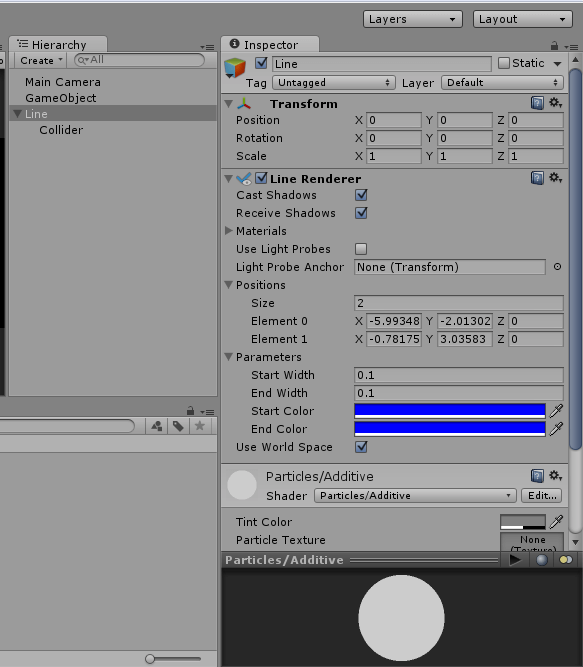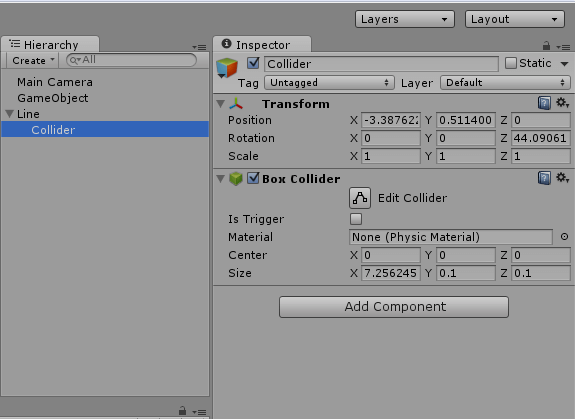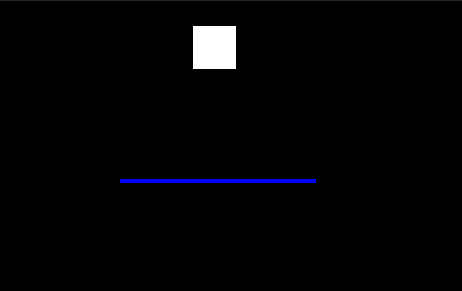## Objective

The objective of this blog post is to explain how to add Collider to Line Renderer or how to draw physics line like “Free Rider” game in unity.

## Step 1 Introduction

The main purpose of posting this blog is to clear all the doubts in previously uploaded blog “Unity – Draw Line on mouse move and detect line collision in Unity 2D and Unity 3D”, regarding how to draw line with collider as in “Free Rider” game.

In previous blog Draw Line on mouse move and detect line collision in Unity 2D and Unity 3D, how line can be drawn on mouse movement using Line Renderer component and detect line collision, is explained. Now here, how to draw physics line like Free Rider game is explained and to accomplish this, we need to add collider to line.

## Step 2 Basic Steps

• Draw a line using line renderer component.
• Make an empty child game object of the line object.
• Now add BoxCollider component to this empty child object.
• Set its size according to the line’s length and width.
• Set its position according to line’s position elements.
• Calculate the angle between first element of line’s position and second element of line’s position and set Z coordinate of eulerAngles of empty child object to this calculated angle.

## Step 3 Example

The following C# script will draw straight line between mouse down and mouse up positions (or touch begin and touch end positions) and add collider on it.

Screenshots:### 3.1 DrawPhysicsLine Script

• Add following script on empty game object.
``````using UnityEngine;
using System.Collections;

public class DrawPhysicsLine : MonoBehaviour
{
private LineRenderer line; // Reference to LineRenderer
private Vector3 mousePos;
private Vector3 startPos;	// Start position of line
private Vector3 endPos;	// End position of line

void Update ()
{

// On mouse down new line will be created
if(Input.GetMouseButtonDown(0))
{
if(line == null)
createLine();
mousePos = Camera.main.ScreenToWorldPoint(Input.mousePosition);
mousePos.z = 0;
line.SetPosition(0,mousePos);
startPos = mousePos;
}
else if(Input.GetMouseButtonUp(0))
{
if(line)
{
mousePos = Camera.main.ScreenToWorldPoint(Input.mousePosition);
mousePos.z = 0;
line.SetPosition(1,mousePos);
endPos = mousePos;
line = null;
}
}
else if(Input.GetMouseButton(0))
{
if(line)
{
mousePos = Camera.main.ScreenToWorldPoint(Input.mousePosition);
mousePos.z = 0;
line.SetPosition(1,mousePos);
}
}
}
// Following method creates line runtime using Line Renderer component
private void createLine()
{
line.SetVertexCount(2);
line.SetWidth(0.1f,0.1f);
line.SetColors(Color.black, Color.black);
line.useWorldSpace = true;
}
// Following method adds collider to created line
{
BoxCollider col = new GameObject("Collider").AddComponent<BoxCollider> ();
col.transform.parent = line.transform; // Collider is added as child object of line
float lineLength = Vector3.Distance (startPos, endPos); // length of line
col.size = new Vector3 (lineLength, 0.1f, 1f); // size of collider is set where X is length of line, Y is width of line, Z will be set as per requirement
Vector3 midPoint = (startPos + endPos)/2;
col.transform.position = midPoint; // setting position of collider object
// Following lines calculate the angle between startPos and endPos
float angle = (Mathf.Abs (startPos.y - endPos.y) / Mathf.Abs (startPos.x - endPos.x));
if((startPos.y<endPos.y && startPos.x>endPos.x) || (endPos.y<startPos.y && endPos.x>startPos.x))
{
angle*=-1;
}
angle = Mathf.Rad2Deg * Mathf.Atan (angle);
col.transform.Rotate (0, 0, angle);
}``````

I hope you find this blog very helpful while adding collider to Line renderer in Unity. Let me know in comment if you have any questions regarding Unity. I will reply you ASAP.

Got an Idea of Game Development? What are you still waiting for? Contact us now and see the Idea live soon. Our company has been named as one of the best Game Development Company in India.I am professional Game Developer, developing games in cocos2d(for iOS) and unity(for all platforms). Games are my passion and I aim to create addictive, high quality games. I have been working in this field since 1 year.